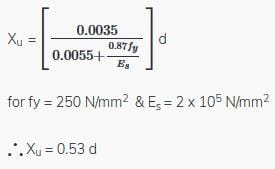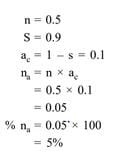Test: B.M.C Soil,Concrete- 2

# Test: B.M.C Soil,Concrete- 2

Test Description

## 25 Questions MCQ Test Civil Engineering SSC JE (Technical) | Test: B.M.C Soil,Concrete- 2

Test: B.M.C Soil,Concrete- 2 for Civil Engineering (CE) 2022 is part of Civil Engineering SSC JE (Technical) preparation. The Test: B.M.C Soil,Concrete- 2 questions and answers have been prepared according to the Civil Engineering (CE) exam syllabus.The Test: B.M.C Soil,Concrete- 2 MCQs are made for Civil Engineering (CE) 2022 Exam. Find important definitions, questions, notes, meanings, examples, exercises, MCQs and online tests for Test: B.M.C Soil,Concrete- 2 below.
Solutions of Test: B.M.C Soil,Concrete- 2 questions in English are available as part of our Civil Engineering SSC JE (Technical) for Civil Engineering (CE) & Test: B.M.C Soil,Concrete- 2 solutions in Hindi for Civil Engineering SSC JE (Technical) course. Download more important topics, notes, lectures and mock test series for Civil Engineering (CE) Exam by signing up for free. Attempt Test: B.M.C Soil,Concrete- 2 | 25 questions in 50 minutes | Mock test for Civil Engineering (CE) preparation | Free important questions MCQ to study Civil Engineering SSC JE (Technical) for Civil Engineering (CE) Exam | Download free PDF with solutions
 1 Crore+ students have signed up on EduRev. Have you?
Test: B.M.C Soil,Concrete- 2 - Question 1

### The shape of idealized stress-strain curve for concrete as prescribed by IS 456-1978 is:

Test: B.M.C Soil,Concrete- 2 - Question 2

### In limit state design, the limiting value of depth of neutral axis for M15 & fe 250 is

Detailed Solution for Test: B.M.C Soil,Concrete- 2 - Question 2Test: B.M.C Soil,Concrete- 2 - Question 3

### Deflection can be controlled by using the appropriate:

Test: B.M.C Soil,Concrete- 2 - Question 4

In the limit state design of concrete structures, the strain distribution is assumed to be:

Test: B.M.C Soil,Concrete- 2 - Question 5

For slabs spanning in two directions for calculating the span to effective depth ratio:

Test: B.M.C Soil,Concrete- 2 - Question 6

The clear distance between the lateral restraints for a simply supported or continuous beam toensure lateral stability should not exceed:

Test: B.M.C Soil,Concrete- 2 - Question 7

In doubly reinforced sections, total reinforcement percentage of steel should not exceed:

Detailed Solution for Test: B.M.C Soil,Concrete- 2 - Question 7

Test: B.M.C Soil,Concrete- 2 - Question 8

A simply supported beam is considered as a deep beam if the ratio of effective span to overall depth is less than:

Test: B.M.C Soil,Concrete- 2 - Question 9

The term ‘Characteristic load’ means that load which has a probability of not being exceeded,during the life of the structure is equal to:

Test: B.M.C Soil,Concrete- 2 - Question 10

For reinforced concrete members totally immersed in sea water, the additional cover thickness recommended by the code is:

Test: B.M.C Soil,Concrete- 2 - Question 11

If the soil sample is having porosity 50% and degree of saturation at 90% then its percentage air voids is:

Detailed Solution for Test: B.M.C Soil,Concrete- 2 - Question 11Test: B.M.C Soil,Concrete- 2 - Question 12

The commonly used method for quick determination of water content at field embankments is:

Test: B.M.C Soil,Concrete- 2 - Question 13

The toughness index is define as the ratio of:

Test: B.M.C Soil,Concrete- 2 - Question 14

According to Atterberg, the soil is said to be of medium plasticity if the plasticity index (PI) is:

Test: B.M.C Soil,Concrete- 2 - Question 15

A soil has liquid limit 65%, plastic limit of 30% and shrinkage limit of 25%, and it has natural moisture content of 45%. The liquidity index of soil is.

Detailed Solution for Test: B.M.C Soil,Concrete- 2 - Question 15

Liquidity index = (water content-plastic limit)/(liquid limit-plastic limit).
= (45-30)/(65-30)
= 15/35
= 0.43

Test: B.M.C Soil,Concrete- 2 - Question 16

Void ratio of a soil is 0.9 its porosity shall be:

Test: B.M.C Soil,Concrete- 2 - Question 17

A soil has percentage air voids of the order of 30%. It has a porosity of 0.4 The air content of that soil shall be: -

Test: B.M.C Soil,Concrete- 2 - Question 18

The ratio of plasticity index and flow index of soil is called:

Test: B.M.C Soil,Concrete- 2 - Question 19

Plasticity chart gives a relation between:

Test: B.M.C Soil,Concrete- 2 - Question 20

A negative value of the group index of a soil is reported as:

Test: B.M.C Soil,Concrete- 2 - Question 21

A soil with a plasticity index equal to zero may be called as:

Test: B.M.C Soil,Concrete- 2 - Question 22

The meniscus and dispersing agent corrections, in the hydrometer analysis, respectively are

Test: B.M.C Soil,Concrete- 2 - Question 23

An inorganic clay of high compressibility is represented by the symbol

Test: B.M.C Soil,Concrete- 2 - Question 24

If void ratio is 0.67, water content = 0.188 and Sp. Gr. = 2.68, the degree of saturation of soil is

Test: B.M.C Soil,Concrete- 2 - Question 25

A sample of saturated clay has a porosity of 0.562, the void ratio of the clay is

## Civil Engineering SSC JE (Technical)

2 videos|111 docs|50 tests
 Use Code STAYHOME200 and get INR 200 additional OFF Use Coupon Code
Information about Test: B.M.C Soil,Concrete- 2 Page
In this test you can find the Exam questions for Test: B.M.C Soil,Concrete- 2 solved & explained in the simplest way possible. Besides giving Questions and answers for Test: B.M.C Soil,Concrete- 2, EduRev gives you an ample number of Online tests for practice

## Civil Engineering SSC JE (Technical)

2 videos|111 docs|50 tests